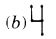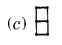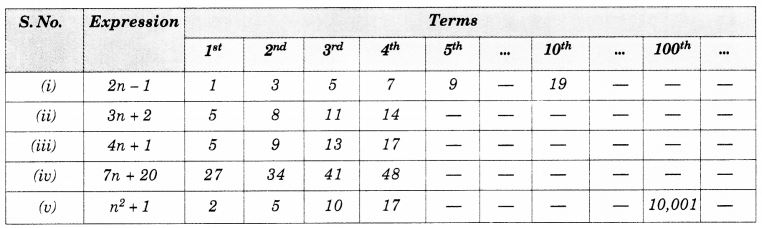NCERT Solutions for Class 7 Maths Chapter 12 Algebraic Expressions Ex 12.4 are part of NCERT Solutions for Class 7 Maths. Here we have given NCERT Solutions for Class 7 Maths Chapter 12 Algebraic Expressions Ex 12.4.

 Board CBSE Textbook NCERT Class Class 7 Subject Maths Chapter Chapter 12 Chapter Name Algebraic Expressions Exercise Ex 12.4 Number of Questions Solved 2 Category NCERT Solutions

## NCERT Solutions for Class 7 Maths Chapter 12 Algebraic Expressions Ex 12.4

Question 1.
Observe the patterns of digits made from line segments of equal length. You will find such segmented digits on the display of electronic watches or calculators.If the number of digits formed is taken to be n, the number of segments required to form n digits is given by the algebraic expression appearing on the right of each pattern.
How many segments are required to form 5, 10, 100 digits of the kindSolution:Let the number of digits formed be n, Then, the number of segments required to form n digits is given by the algebraic expression 5n + 1.
So,

1. the number of segments required to form 5 digits of this kind = 5 × 5 + 1 = 25 + 1 = 26
2. the number of segments required to form 10 digits of this kind = 5 × 10 + 1 = 50 + 1 = 51
3. the number of segments required to form 100 digits of this kind = 5 × 100 + 1 = 500 + 1 = 501.Let the number of digits formed be n. Then, the number of segments required to form n digits is given by the algebraic expression 3n + 1.
So,

1. the number of segments required to form 5 digits of this kind = 3 × 5 + 1 = 15 + 1 = 16
2. the number of segments required to form 10 digits of this kind = 3 × 10 + 1 = 30 + 1 = 31
3. The number of segments required to form 100 digits of this kind = 3 × 100 + 1 = 300 + 301.Let the number of digits formed be n. Then, the number of segments required to form n digits is given by the algebraic expression 5n + 2.
So,

1. the number of segments required to form 5 digits of this kind = 5 × 5 + 2 = 25 + 2 = 27
2. the number of segments required to form 10 digits of this kind = 5 × 10 + 2 = 50 + 2 = 52
3. the number of segments required to form loo digits of this kind = 5 × 100 + 2 = 500 + 2 = 502.

Question 2.
Use the given algebraic expression to complete the table of number patterns.Solution:We hope the NCERT Solutions for Class 7 Maths Chapter 12 Algebraic Expressions Ex 12.4 help you. If you have any query regarding NCERT Solutions for Class 7 Maths Chapter 12 Algebraic Expressions Ex 12.4, drop a comment below and we will get back to you at the earliest.